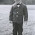## Wednesday, March 20, 2013

### Wednesday Brain Teaser 3-20-13

What's so special about the number 2520?

#### 6 comments:

1.Nobody got that one yet? It isn't fair for me to answer--I assigned that problem (the inverse) when homeschooling math, so I recognize it.

1.Your ethics in recusing yourself are admirable.

2.Let's see...2520 is divisible by 9. (The digits sum to a value divisible by 9.)
And divisible by 10, 5. (Last digit is zero.)
And divisible by 8. (Since 520 is divisible by 8.)
And divisible by 7. (A little harder...used this algorithm. Also got 2520/7 = 360.)

Divisibility by 9 implies divisibility by 3.
Divisibility by 8 implies divisibility by 2 and 4.
Both of the above imply divisibility by 6.

Thus, 2520 is divisible by 2, 3, 4, 5, 6, 7, 8, 9, and 10. (And divisible by 1.)

Looking at the factorization, 2520 = 5(7)8(9) = 2^3(3^2)5(7), so there shouldn't be a smaller integer divisible by all integers in the range [1,2,...10].

1.Full credit for showing your work.

3.Mathematics is not my strong point with the exception of giving birth to a mathematician. My initial thought was "But... it was 2525 wasn't it?"

4.Sweet. SJ March, I'm handing this to my 15 year old. heh. heh. heh.

I followed the process until you looked at factorization. I understand your conclusion but not the process there. Don't tell me. I guess I will have to have my 15 year old explain it to me.

They say that teaching someone else helps you learn. heh. heh. heh.